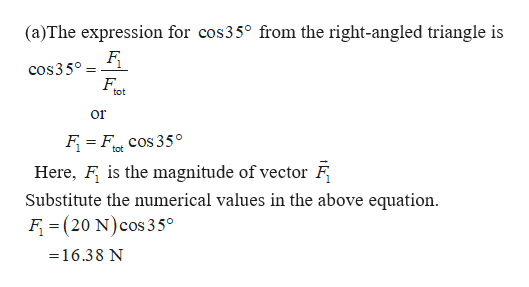Question
3 views

Consider the forces shown in the figure.

a.) Find the magnitude of the force F1 shown in the figure in Newtons.

b.) Find the magnitude of the force F2 shown in the figure in Newtons.

check_circle

Step 1help_outlineImage Transcriptionclose(a)The expression for cos35° from the right-angled triangle is cos350 F tot Or tot Here, F is the magnitude of vector F Substitute the numerical values in the above equation F(20 N) cos 35° =16.38 N fullscreen

### Want to see the full answer?

See Solution

#### Want to see this answer and more?

Solutions are written by subject experts who are available 24/7. Questions are typically answered within 1 hour.*

See Solution
*Response times may vary by subject and question.
Tagged in

### Vectors and Scalars